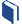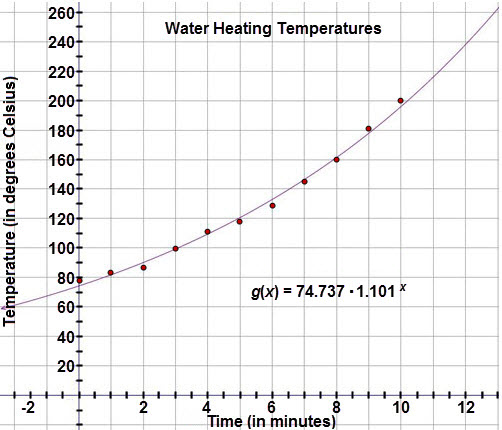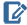# A Good Enough Fit?

###Resources for this lesson:

You will use your Algebra II Journalon this page.

> Glossary> Calculator Resources> Teacher Resources: Instructional NotesMarissa drew the exponential curve of best fit on the scatter plot, as seen below:## Test and Confirm

For the purposes of this course, the correlation coefficient should only be analyzed with linear models.  Since this model is exponential, Marissa will only need to analyze the residual plot.

After examining the graph, Marissa believes that the exponential model is the best fit for the data and is more confident with her predictions.

##Algebra II Journal: Reflection 3

In this lesson, you learned how to analyze a fit for a data set using residuals and, for linear models, correlation coefficient.  Respond to the following reflection questions in your Algebra II Journaland submit to your teacher.

• Calculate and plot the residuals for the exponential model of best fit.  Is Marissa making a reasonable conclusion that an exponential model is a good fit for her data?  Why or why not?
• How can you determine if a linear regression equation is the best fit for a data set?
• What are residuals?  How can we use residuals to analyze the fit for a model?